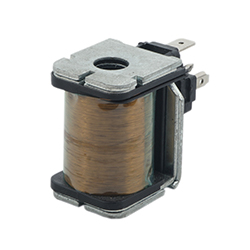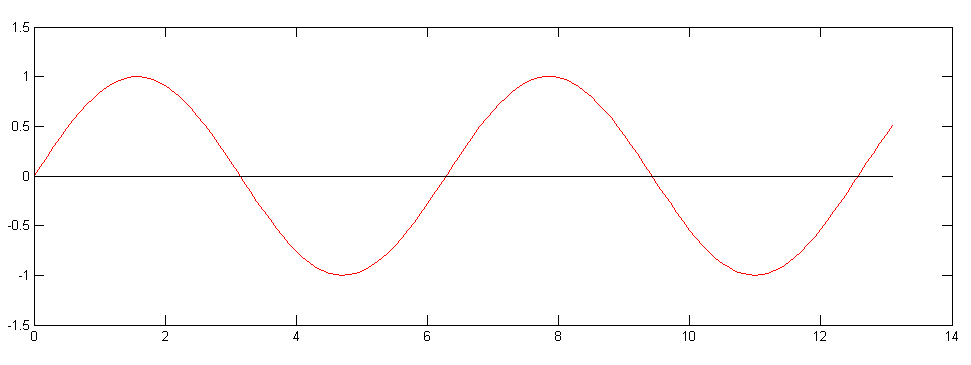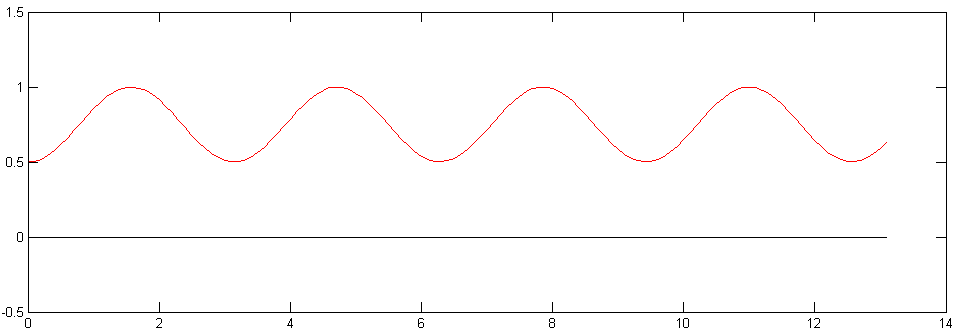# Choosing an AC or DC solenoid valve coilFigure 1: Solenoid valve coil

## Solenoid working principles

Solenoids are the most important components used in solenoid valves to control the flow of liquids and gases. Solenoids are electromechanical devices that convert AC or DC electrical energy into linear motion. They usually consist of a helical coil wound concentrically around a movable cylinder, called the armature, made from a ferromagnetic material such as iron or steel. Most solenoid valves have a replaceable coil and can be used with coils with different voltages.

When current flows through the coil, it generates a magnetic field inside the coil which attracts the armature towards the center of the solenoid using the same basic principles as ordinary electromagnets. Since the armature is drawn towards the center of the solenoid regardless of the polarity of the current, an opposing force is needed to return the armature into the starting position when the coil is not energized. This is achieved by using a spring mechanism. Under ideal conditions, in order to actuate the solenoid, the force generated by the solenoid must be larger than the combined forces of the spring, and the hydraulic pressure, as well as friction.

By lifting the armature, a small port in the valve is opened that allows the flow of the media. The flow through the valve can be controlled by energizing or de-energizing the coil. While there are several types of solenoid valves which differ in their mechanical construction , the basic idea of a solenoid actuator acting on a control surface remains the same in all solenoid valve types.

The polarity of the electrical contacts is not important with AC and DC solenoid valves. With AC solenoid valves, this might be obvious because the current switches polarity twice per period anyway. With DC solenoid valves the reasoning is that current passing through the coil creates an electromagnet which produces an attractive force on the armature. When current is applied through the coil, the armature will always be pulled towards the coil, regardless of the contact and current polarity.

## Differences between AC and DC solenoids

At the most basic level, the operation of DC solenoids is relatively straightforward - the solenoid may be energized, allowing the magnetic force generated by the solenoid to overcome spring resistance and moving the armature towards the center of the coil, or de-energized, allowing the spring force to push the armature back to the starting position.

With AC solenoids, the theory of operation is slightly more complicated. AC current can be approximated using a sinusoidal waveform. As a consequence, twice per period the current has a zero-crossing, meaning that the current flowing through the coil at that point in time is equal to zero.Since the magnetic force generated by the solenoid is in direct proportion to the current flowing through the solenoid coil, the spring force will overcome the force generated by the solenoid for a short period of time, twice per period. This is a problem which manifests as a vibration of the armature, which produces a humming sound and can cause stress on solenoid valve components. To avoid this issue, a simple conductive ring, termed a shading ring, is installed near the coil around the armature. The shading ring is usually made from copper. The function of a shading ring is to store magnetic field energy and release it with a 90 degree phase difference.The effect of a shading ring is that while the magnetic field generated by the primary coil decreases towards zero, the magnetic field generated by the shading ring peaks, effectively filling in the gap in the magnetic field amplitude during zero crossings, eliminating the vibrations. Most solenoid valves that can be used with different coil voltages have a built-in shading ring.

If dirt collects around the armature, the effect of the shading ring may be limited and another solution is required. An example of another solution is the use of an electronic circuit which filters the solenoid current, so that there are no zero-crossings. This circuitry can be embedded into the solenoid valve coil itself or it can be built externally. It is usually implemented using rectifier diodes and a filtering capacitor in a full-wave rectifier topology.

## Using AC coils with DC current and vice versa

In some cases, coils rated for AC current can be used with DC power supplies and vice versa. However, there are some limitations to keep in mind.

Using a coil rated for AC current with a DC supply is possible, but the voltage (and the current) must be limited or else the solenoid might burn out. The reason for this is that in AC regime, coils have an inductive reactance that adds up with the electrical resistivity of the coil. As a result, the impedance of a coil is several times higher in AC regime than in DC regime. As an example, using a 24 VAC rated solenoid valve with a 24 V DC power supply would most likely damage the solenoid because the effective current flowing through the solenoid would be much higher with DC voltage.

Unfortunately, there is no fixed factor for derating the power supply voltage. The effective current should be measured in AC regime, and that current should be set as a target for DC regime as well. Some ways to achieve that target would be reducing the supply voltage or using a current-limiting resistor.

Using a coil rated for DC current with an AC power supply, imposes the risk of vibrations since DC solenoid valves might not contain a shading ring or a rectifier circuit. These vibrations might damage the solenoid by stressing the components over time, and they can contribute to noise levels in the room. This can be worked around by using an external full-wave rectifier circuit with a capacitive filter.

Another problem is that the effective current will be several times lower in this case, and the magnetic force generated by the coil might not be large enough to move the armature from its resting position. A solution would be to use a larger voltage so that the effective current matches the rated current of the solenoid.

## AC vs. DC solenoid design considerations

Ideally, when a solenoid valve goes from OFF to ON state, the solenoid should initially generate more force in order to overcome spring tension combined with hydraulic pressure acting against the valve. Once flow is established, hydraulic forces acting on the valve mechanism decrease, and the solenoid can decrease the generated force in order to reduce power consumption and heating.

AC solenoids follow this ideal behaviour more closely than DC solenoids. In DC solenoids, when the solenoid is turned on, the current rises asymptotically towards a certain value depending on the resistivity of the coil. This translates to a lower initial current (and lower initial force leading to slower valve opening). Once the valve is open, the current draw remains at a constant value which is larger than needed to keep the valve open. As a result, DC solenoids without any external circuitry will waste a considerable amount of power in the open state.

For AC circuits, the impedance of a coil is calculated using the following formula:In the above formula Z is the impedance, R is the electrical resistance of the coil, j is a constant equal to the square root of -1 which in this equation has an effect of shifting phase by 90 degrees, f is the frequency and L is the inductance of the coil. At first, the air gap is large and as a result, the coil inductance is small, leading to a smaller impedance and a larger current through the solenoid. Larger current equals higher magnetic force on the armature.

As the valve opens, the air gap becomes smaller and smaller and the impedance of the coil increases rapidly, decreasing the current through the coil. Reduced current through the coil results in reduced power consumption and wasted heat. Because of this, AC solenoids generate an initial spike in current, which enables a quicker, more powerful opening of the valve. As soon as  the valve is open, the current drops, which reduces the power consumption.

Although AC solenoids are inherently more energy efficient, they come with some possible drawbacks. One of them is power loss due to Eddy currents which form due to electromagnetic induction in the armature. Another drawback is the risk of vibration, which can be mitigated by using well-engineered solenoid valves which make use of appropriate shading rings. In addition, modern control systems tend to provide easier interfacing with DC outputs, so using AC solenoids with these systems can be more cumbersome and require the use of additional relays.

DC solenoids can be made more efficient by using external circuits which can shape the coil current in such a way that there is an initial current spike required to open the valve. Once the valve opens, the current can be reduced to a maintenance current level, which is just enough to reliably keep the valve open by pulling the armature against the spring tension.

These external circuits can be as simple as connecting the coil in series with a parallel connection of a resistor and a capacitor. In such a circuit, charging the capacitor through the coil provides an initial coil current spike. After the capacitor is charged, the current-limiting resistor passes all the current. The downside of such a simplistic approach is that some of the power is wasted on heating the current-limiting resistor.

There are much more complex approaches involving switched power supplies which provide a programmable current to the coil. These power supplies may work with both AC and DC solenoid valves and power supplies. They ensure a good valve opening spike and reduced power consumption while the valve is open, resulting in better power efficiency, less heating and prolonged solenoid valve service life.